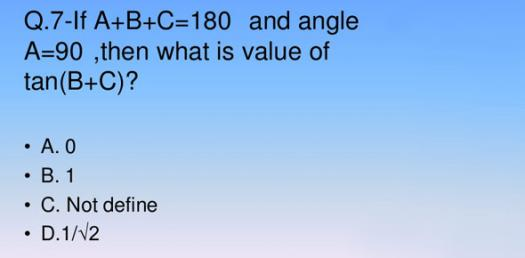# Math General Knowledge Questions: Quiz!

13 Questions | Total Attempts: 811SettingsCreate your own QuizMathematical equations can be very interesting if you get to know the concept behind them and the different formulas for solving them. The quiz below offers you some review materials that will help you in your final exam this year. Give it a shot and all the best in your upcoming exams.

• 1.
What is sin 34 degrees (rounded to 4 places)?
• A.

0.5591

• B.

0.5594

• C.

0.5593

• D.

0.5592

• 2.
If 1/6 + 1/7 + 1/8 > 1/x + 1/7 + 1/8, then x could have which of these values?
• A.

3

• B.

6

• C.

7

• D.

4

• 3.
What is an equation of the line that passes through point (3,0) and point (0,-3) ?
• A.

Y=x+3

• B.

Y=3x

• C.

X=y+3

• D.

Y=x-3

• 4.
If z is a positive integer divisible by 7 and z
• A.

90

• B.

87

• C.

84

• D.

82

• 5.
What is the derivative of (x+2)^2 with respect to x ?
• A.

4x+4

• B.

X+2

• C.

2x^2+4x+4

• D.

2x+4

• 6.
In the equation 4x - 3 / 9x + 4 = 1 2/7 what does "X" equal?
• A.

X = -1 4/12

• B.

X = 1 4/53

• C.

X = -1 12/34

• D.

X = -1 3/12

• 7.
If x=2, what is y in 3x - 4y = 10 ?
• A.

Y = 1

• B.

Y = -1

• C.

Y = 4

• D.

Y = -4

• 8.
In the equation 3x + 1 / 2x + 3 = 2 1/2, what does "x" equal?
• A.

X = -2 6/8

• B.

X = -3 1/2

• C.

X = -4 5/8

• D.

X = -3 1/4

• 9.
What is the solution of X^2 + 4*X + 4 = 0 ?
• A.

X=-4

• B.

X=2

• C.

X=-2

• D.

X=2 Or X=-2

• 10.
How many real roots does the polynomial x^4+x^2+2 have?
• A.

1

• B.

4

• C.

0

• D.

2

• 11.
If x, y and z are positive integers and (a - b)c = 0, which statement must be true?
• A.

B = c

• B.

B > a

• C.

A < c

• D.

A = b

• 12.
How many of the prime factors of 60 are greater than 2?
• A.

Zero

• B.

One

• C.

Two

• D.

Five

• 13.
Who is known as the father of geometry?
• A.

Newton

• B.

Fermat

• C.

Pascal

• D.

Euclid

Related TopicsBack to top
×

Wait!
Here's an interesting quiz for you.